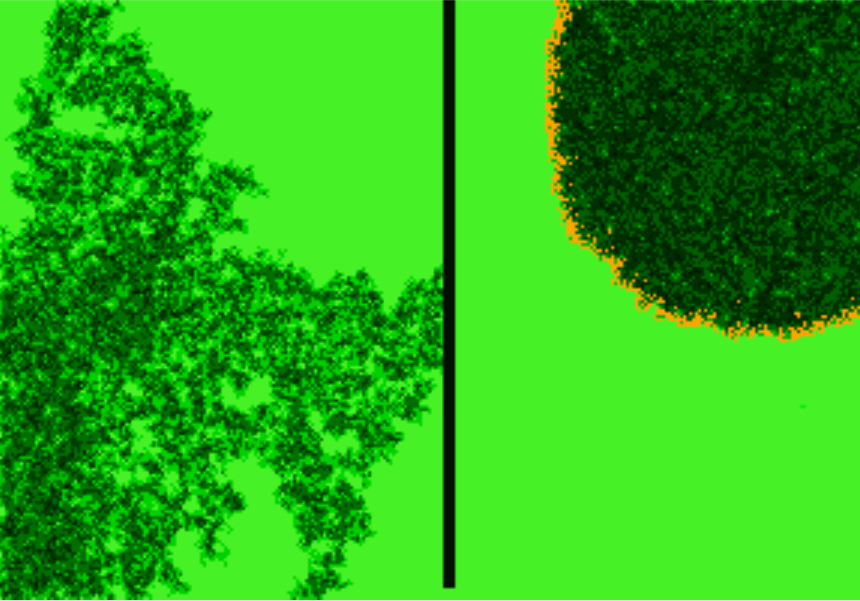# biomass

## Fire spread -- rectangular grid

ModelId:
SimileVersion:
6.6

This is a very simple model of fire spread in a patch of vegetation. The model consists of a rectangular array of grid squares, created using the rectangular array built-in type. Vegetation in each square is modlled by a single compartment with a growth function that produces a sigmoid curve in the absence of any burning.

Equations:

Model Desktop1 :

Variable   doing fire :
doing fire =         any(any([[lit]])) (boolean)
Where:

True if any square i the grid is on fire. Prevents normal growth or spontaneous ignition from happening (because timescale is shorter)

Variable   done fire :
done fire =         last(doing_fire) (boolean)
Where:
doing_fire = Value(s) of doing fire

Take the last value of doing_fire here -- because using last(doing_fire) inside the submodel will cause an intermediate variable to be created for it in each instance, which wastes memory.

Submodel "Quads" is a fixed_membership multi-instance submodel with dimensions [200,300].
Each instance represents a square area of forest
Enumerated types: {rect_nbr sw s se w e nw n ne}

Compartment   fuel :
Initial value = if firebreak then 0 else 4 (real)
Where:
firebreak = Value(s) of firebreak

Because growth rate is a function of current load, setting the initial value in the firebreak area to zero ensures that it remains at zero.

Flow   burn :
burn =         if lit then 0.5*fuel/dt() else 0 (1/day)
Where:
fuel = Value(s) of fuel
lit = Value(s) of lit

Half the fuel in a cell will be consumed if it catches; it might catch more than once in a single fire, causing uneven burn patterns.

Flow   growth :
growth =         if doing_fire then 0 else 0.001*fuel*(100-fuel) (1/day)
Where:
fuel = Value(s) of fuel
doing_fire = Value(s) of ../doing fire

This function causes the fuel load to follow a sigmoid curve levelling out at 100 in the absence of fire. Fires happen much quicker than growth, so growth is set to zero while a fire is burning because model time takes on a different meaning.

Variable   firebreak :
firebreak =         column_id()>148 and column_id()<=152 and row_id()>4 (boolean)

Incomplete firebreak down the middle of the simulated area.

Variable   lit :
lit =         not done_fire and luck*1e8<fuel or luck*200<fuel*last(nbrs_lit) (boolean)
Where:
nbrs_lit = Value(s) of nbrs lit
fuel = Value(s) of fuel
luck = Value(s) of luck
done_fire = Value(s) of ../done fire

A cell will burn if its neighbours were burning last time step and it is unlucky, or if nothing was burning last time step and it is very unlucky (e.g., gets hit by lightning). More neighbours alight means more chance of burning. There must be more fuel available than luck!

Variable   luck :
luck =         rand_var(0,1) (real)

Adds the random element; the lower it is, the more likely a cell is to burn.

Variable   nbrs lit :
nbrs lit =         howmanytrue({from_8_nbrs_lit}) (int)
Where:
{from_8_nbrs_lit} = Value(s) of lit
lit = Value(s) of lit

Counts the number of a cell's eight neighbours which are alight.

Variable   show :
show =         if lit then -3 else fuel (real)
Where:
fuel = Value(s) of fuel
lit = Value(s) of lit

The one on the right is currently burning and covering a circular area because fuel is abundant.